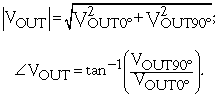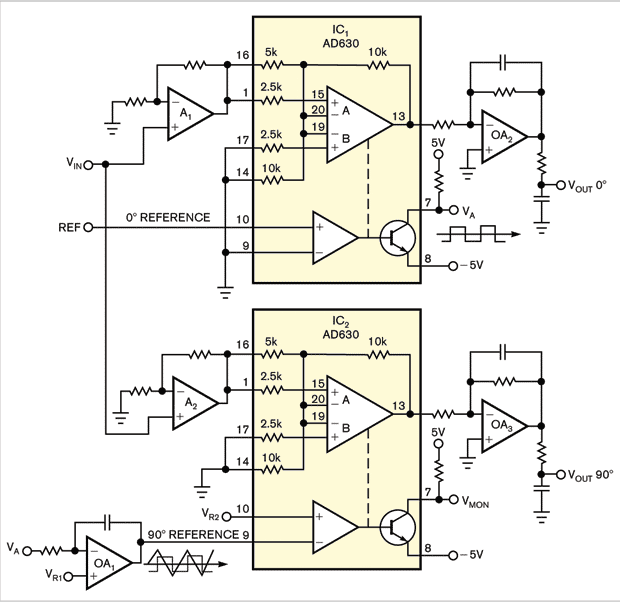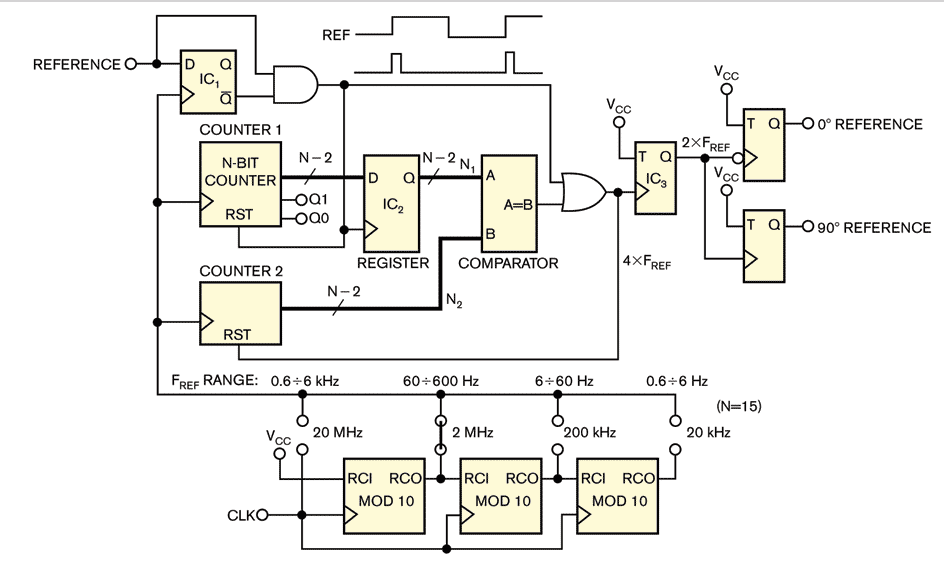# Compact, four-quadrant lock-in amplifier generates two analog outputs

Generate a pair of quadrature-phase digital signals.

Stefano Salvatori and Marco Girolami

The circuit in this Design Idea realizes a simple, low-cost lock-in amplifier employing an Analog Devices AD630 balanced modulator-demodulator IC (Reference 1). The device uses laser-trimmed thin-film resistors, yielding accuracy and stability and, thus, a flexible commutation architecture. It finds use in sophisticated signal-processing applications, including synchronous detection. The amplifier can detect a weak ac signal even in the presence of noise sources of much greater amplitude when you know the signal’s frequency and phase.

As an analog multiplier, the AD630 reveals the component of the input-voltage signal in a narrow band around the frequency of the reference signal. The lowpass filter at the AD630’s output allows you to gain information on the weak signal amplitude, which the uncorrelated noise originally masked. When the input voltage and the reference voltage are in phase, the lowpass filter’s output, VOUT, assumes the maximum amplitude. Conversely, if the input voltage and the reference voltage are in quadrature, the output voltage would ideally be 0V. In this way, if both in-phase and quadrature reference signals are available, two balanced demodulators reveal the in-phase output voltage to be 0° and the in-quadrature output voltage to be 90°. You can calculate the module and phase shift as follows:The two AD630s have a gain of ±2 and receive the amplified signal, VIN, through two identical amplifiers, A1 and A2. At Pin 7 of IC1, a bipolar ±5V squared signal appears in phase with the reference signal. OA1 integrates the amplifier voltage, which generates a triangular wave that IC2’s comparator compares with the VR2 voltage. You must regulate VR1 and VR2 to obtain a perfect 90°-shifted command for IC2. You can monitor the voltage at IC2’s Pin 7. Measurement accuracy and repeatability depend strongly on the RC time constant of the integrator and the values of VR1 and VR2.Figure 1. OA1 integrates the bipolar VA signal and creates a triangular wave. VR1 and VR2 obtain a 90°-shifted reference voltage with respect to VA.

You can use a different approach to generate in-phase and in-quadrature reference signals. Figure 2 shows an all-digital circuit, which you can implement in a small CPLD (complex programmable-logic device) to generate the 0 and 90° reference signals in Figure 1. Counter 1 measures the reference-signal time in terms of the N number of digital clock pulses, where the reference time can be different from 50%. It receives a preset command at the N1=1 value at each positive front edge of the reference signal. D-type flip-flop IC1 generates such pulses. At each positive edge of the reference signal, IC2 acquires the N/4 value. Meanwhile, Counter 2 counts the clock periods and receives a restart command at the N2=1 value when its value reaches the comparator-measured N/4 quantity.Figure 2. You can implement this all-digital circuit in a small CPLD

To overcome the lack of the last EQ signal when the reference time is greater than approximately four times the N/4 integer value, the OR combination of the two RST and EQ pulses yields four almost-equidistant positive-edge commands in each reference-time period. The N/4 integer division, a logical right shift by 2 bits of N1, gives a maximum error of three on the last pulse position. These pulses generate the in-phase and in-quadrature signals, 0 and 90°, respectively, resulting from simple commutations on the positive or negative edges of the signal. T-type flip-flop IC3 generates a signal with twice the frequency of the reference signal. In this way, the accuracy is equal to 3/N1.

To maintain accuracy at least comparable with that of the AD630, the N1 output of Counter 1 would be the highest. However, an increase in the number of bits decreases the maximum reference frequency for a given digital-clock frequency if you want N1 to reach high values. For example, if N is 15 bits, the N1 output assumes the 32,767 maximum value with an accuracy of approximately 0.01%. If the reference-time period decreases, you can assume a minimum value of 3277—that is, one-tenth of the maximum value—for N1, with a correspondingly lower accuracy of 0.1%, which is comparable to the gain accuracy of the AD630. To increase the reference frequency, divide the digital clock’s frequency to select low values when the reference time becomes too long.

EDN